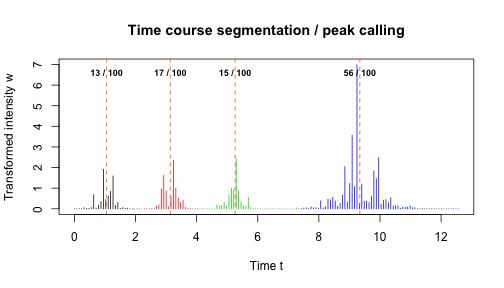This tutorial illustrates applications of optimal univariate clustering function Ckmeans.1d.dp. It clusters univariate data given the number of clusters $$k$$. It can estimate $$k$$ if not provided. It can also perform optimal weighted clustering when a weight vector is provided with the input univariate data. Weighted clustering can be used to analyze 1-D signals such as time series data. The corresponding clusters obtained from weighted clustering can be the basis for optimal time course segmentation or optimal peak calling.

1. Cluster univariate data given the number of clusters $$k$$

Example 1.

Cluster data generated from a Gaussian mixture model of three components.

The number of clusters is provided.

require(Ckmeans.1d.dp)

## Loading required package: Ckmeans.1d.dp

x <- c(rnorm(50, sd=0.3), rnorm(50, mean=1, sd=0.3), rnorm(50, mean=2, sd=0.3))
# Divide x into 3 clusters
k <- 3
result <- Ckmeans.1d.dp(x, k)
plot(result)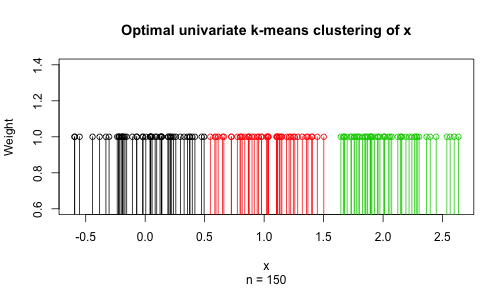plot(x, col=result$cluster, pch=result$cluster, cex=1.5,
main="Optimal univariate clustering given k",
sub=paste("Number of clusters given:", k))
abline(h=result$centers, col=1:k, lty="dashed", lwd=2) legend("bottomright", paste("Cluster", 1:k), col=1:k, pch=1:k, cex=1.5, bty="n")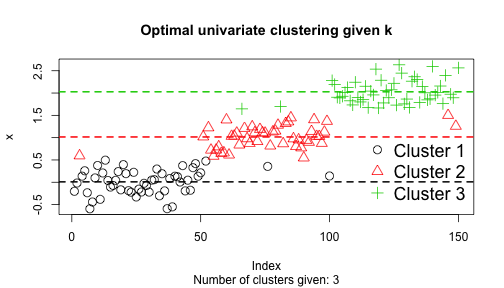2. Cluster univariate data with an estimated number of clusters $$k$$ Example 2. Cluster data generated from a Gaussian mixture model of three components. The number of clusters is determined by Bayesian information criterion: require(Ckmeans.1d.dp) x <- c(rnorm(50, mean=-1, sd=0.3), rnorm(50, mean=1, sd=1), rnorm(50, mean=2, sd=0.4)) # Divide x into k clusters, k automatically selected (default: 1~9) result <- Ckmeans.1d.dp(x) plot(result)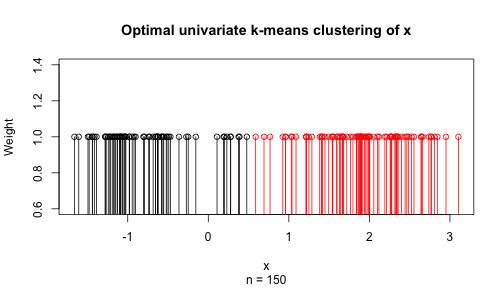k <- max(result$cluster)
plot(x, col=result$cluster, pch=result$cluster, cex=1.5,
main="Optimal univariate clustering with k estimated",
sub=paste("Number of clusters is estimated to be", k))
abline(h=result$centers, col=1:k, lty="dashed", lwd=2) legend("topleft", paste("Cluster", 1:k), col=1:k, pch=1:k, cex=1.5, bty="n")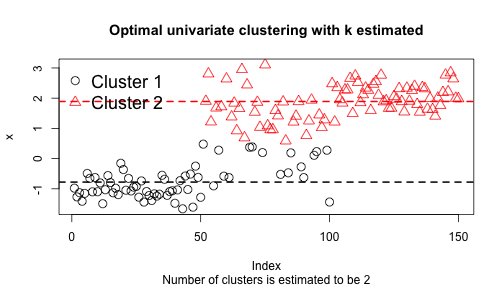3. Optimal weighted univariate clustering for time series analysis Example 3. We segment a time course to identify peaks using weighted clustering. The input data is the time stamp of obtaining each intensity measurement; the weight is the signal intensity. require(Ckmeans.1d.dp) n <- 160 t <- seq(0, 2*pi*2, length=n) n1 <- 1:(n/2) n2 <- (max(n1)+1):n y1 <- abs(sin(1.5*t[n1]) + 0.1*rnorm(length(n1))) y2 <- abs(sin(0.5*t[n2]) + 0.1*rnorm(length(n2))) y <- c(y1, y2) w <- y^8 # stress the peaks res <- Ckmeans.1d.dp(t, k=c(1:10), w) plot(res)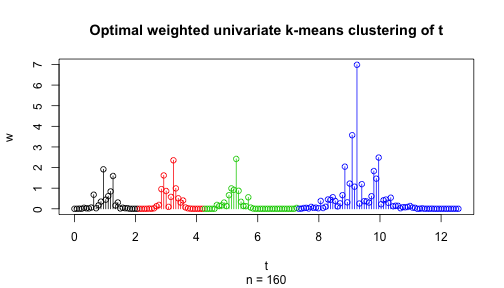plot(t, w, main = "Time course segmentation / peak calling", col=res$cluster, pch=res$cluster, type="h", xlab="Time t", ylab="Transformed intensity w") abline(v=res$centers, col="chocolate", lty="dashed")
text(res$centers, max(w) * .95, cex=0.75, font=2, paste(round(res$size / sum(res\$size) * 100), "/ 100"))